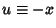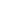## Fourier Transform--Exponential Function

The Fourier Transform ofis given by(1)

Now letso, then(2)

which, from the Damped Exponential Cosine Integral, gives(3)

which is a Lorentzian Function.